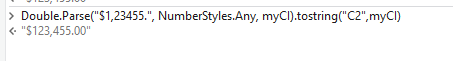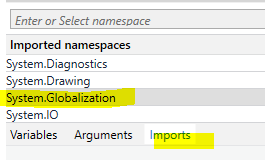# How do you add a decimal in a currency when its not there?

I have a field in my data table called “Total” and in extraction sometimes the value \$1,234.55 gets extracted as \$1,23455

How do I add a decimal if its not there in a value?

@postwick would you be able to help on this?

if the value \$1,23455. is meaning \$123455.00 then give a try on followingwithIn case that
\$1,23455. is meaning \$1234.55 then we would recommend analyzing the source of this value (e.g. from where the datatable is populated), due the shifted . on end cannot be ralized as decimal seperator needed on some other positions

Hi @ppr Thank you for your reply. the value \$1,234.55 is getting extracted as \$1,23455

I dont want a decimal .00 in the end but rather want the result to be \$1,234.55

then the second part of my answer is to target. We would not recommend to shift the . two positions to left depend on its absence as it create risks and side effects

Thank you. How do you recommend to do that?